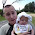## Tuesday, December 20, 2011

### Observing the geometric phase in magnetoresistance II

Previously I posted how one could see a signature of Berry's geometric phase in Shubnikov de Haas experiments on graphene. Similar experiments and analysis of data for topological insulators produces ambiguous results, as discussed in this recent PRB by Taskin and Ando. In particular, the Berry phase gamma can be extracted from a "Landau level fan diagram" where one plots the inverse of the magnetic field B vs. the Landau level index N. Taskin and Ando claim that in the topological insulator the dispersion relation E(k) is non-linear and as a result

1. the fan diagram is non-linear

2. the Berry phase gamma deviates from pi and is a function of B

I am confused by 2. I would have thought that topology would require gamma to be only be pi or 0. This could be checked by looking at the eigenfunctions for the non-linear dispersion. Am I missing something?

The above claims are based on eqn. 8 in the paper. It is derived from the generalised Onsager condition which is a semi-classical condition relating the area of the quantised cyclotron orbit to Landau level index N.

I welcome clarifications.

#### 1 comment:

1.As nice as holidays are, I've enjoyed catching up on my science blogs.

The definition of Berry curvature after equation 4 is incorrect, I believe.

Nevertheless, my understanding was that the integral of the Berry curvature over a closed manifold is quantised, not some arbitrary surface (defined by a Fermi circle in this case). So I think that equation 4 integrated around the circle in both senses is quantised, or if you turn equation 8 into a lattice theory and integrate over the Brillouin zone, it should be quantised. This is the Chern number.

I may be mistaken and still in holiday mode, but this was my understanding.

It is an interesting paper. My first naive thought was that the small Pi^2/2m term in equation 8 should not contribute to the Berry curvature, as it's diagonal in the surface Hamiltonian, and equation 4 depends only on wavevectors. But when Landau-level physics enters, this expectation becomes incorrect.